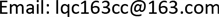192089部队，浙江 宁波

2海军工程大学动力工程学院，湖北 武汉1. 前言

2. 燃料电池(SOFC)与燃气轮机混合系统的顶层循环结构

3. 燃气轮机与燃料电池循环结构的模糊评判模型

∑ i = 1 m U i = U ， { U i ∩ U j = Φ ( i ≠ j ) }

B i = A i ∘ R i = [ b i 1 , b i 2 , ⋯ , b i n ]

R = [ B 1 B 2 ⋮ B m ] = [ b 11 b 12 ⋅ ⋅ ⋅ b 1 n b 21 b 22 ⋅ ⋅ ⋅ b 2 n ⋮ ⋮ ⋱ ⋮ b m 1 b m 2 ⋅ ⋅ ⋅ b m n ]

4. 系统循环结构模糊评判的应用实例

E = { E 1 , E 2 , E 3 , ⋯ , E 16 } = { 0.090 , 0.090 , 0.090 , 0.066 , 0.066 , 0.066 , 0.066 , 0.066 ,         0.060 , 0.060 , 0.060 , 0.044 , 0.044 , 0.044 , 0.044 , 0.044 }

u = { u 1 , u 2 , u 3 , u 4 } = {经济效益，占用体积，寿命，效率}

A = { A 1 , A 2 , A 3 , A 4 } = { 0.23 , 0.15 , 0.11 , 0.51 }

V = { V 1 , V 2 , V 3 , V 4 }

B 1 = A 1 ⋅ R 1 = { 0.16 , 0.25 , 0.23 , 0.27 } B 2 = A 2 ⋅ R 2 = { 0.28 , 0.24 , 0.23 , 0.23 } B 3 = A 3 ⋅ R 3 = { 0.20 , 0.36 , 0.39 , 0.18 } B 4 = A 4 ⋅ R 4 = { 0.36 , 0.31 , 0.21 , 0.12 }

Z = B × F = ( 0.484 , 0.249 , 0.161 , 0.106 ) × ( 90 , 75 , 60 , 50 ) = 77.195

5. 结论